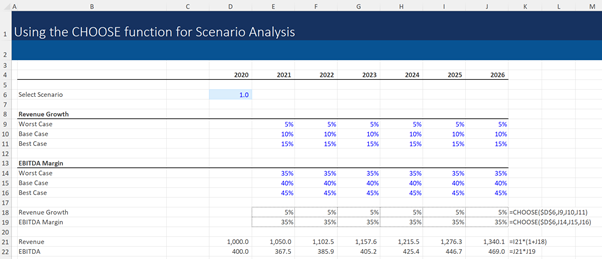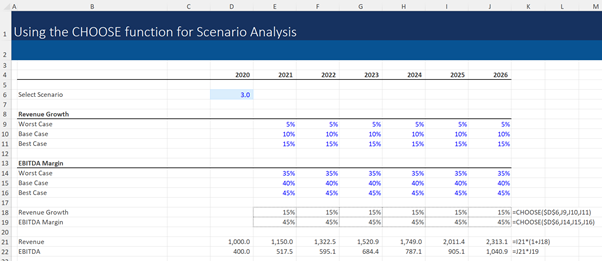## Using Choose Function in Excel

The CHOOSE function is part of a broader set of Microsoft Excel functions that allows analysts to run different scenarios in their model. These functions allow the analyst to flex the assumptions used in the model, making multiple scenario analyses quick and easy.

Financial models will usually, at a minimum, have three sets of assumptions for revenue growth and margins: ‘worst case’, ‘base/median case’ and ‘best case’.  The median case assumptions reflect the most likely expected outcome whilst the worst case and best case usually reflect higher and lower values.

The CHOOSE function allows the user to change which assumptions are used in the model. It works by selecting the index value from a given set of values.

## Choose Function Formula

=CHOOSE(index, value_1, value_2,…)

Where,

Index is the relative position of the value to be selected in the range of values. Index can take values between and including 1 and 254.

Value_1, Value_2, ,.. are the range of values from which desired value is to be selected. The range can have a maximum of 255 values.

In the context of scenario analysis, the index value is usually the scenario number whilst the values are the assumptions for each of the scenarios. For a model with 3 scenarios this would be:

=CHOOSE(scenario number, growth assumption for scenario 1, growth assumption for scenario 2, growth assumption for scenario 3).

The growth assumption used in this model would then depend on the scenario number input by the user.

## Key Learning Points

• Scenario analysis allows analysts to run different scenarios in their model by flexing the input assumptions. Analyst models usually include assumptions for a worst-case, base case, and best case scenario
• The CHOOSE Excel function automates the process of changing the assumptions used during scenario analysis. The user toggles automatically between the scenarios by changing the scenario number used in the model
• The CHOOSE function is simple to understand but can be time-consuming to use if creating many scenarios. It is also inflexible if the user wants to add additional scenarios.

## Why do financial analysts use scenario analysis?

The use of scenario analysis is particularly important in financial modeling because the assumptions made to project future outcomes are not guaranteed. Scenario analysis allows analysts to ‘stress test’ their predictions by demonstrating how the model outputs change when the model assumptions increase or decrease.

Although terminology differs by application and geography, the ‘worst case’ is also known as the ‘bear case’, ‘best case’ is known as the ‘bull case’ and the ‘median case’ also known as the ’base case’. The median case assumptions reflect the analyst’s or management’s expected outcome whilst the bull and bear case usually reflect higher and lower revenue growth and margin assumptions. The bull and bear cases are considered less likely scenarios but should still reflect plausible scenarios.

## When is the CHOOSE function used?

The CHOOSE function is widely used by analysts when running scenario analysis in their models as it is simple to use and understand.

However, it is most widely used when the analyst only needs to run a small number of scenarios. The CHOOSE function requires more keystrokes than alternative Microsoft Excel functions; each value in the formula is manually input and separated by a comma and it can therefore be very time-consuming to use this function if there are more than 3 scenarios.

A further limitation of this function is that the formula is inflexible if the analyst wishes to add extra scenarios; the analyst would need to manually amend the formula by inserting a comma and the appropriate assumption.

## Excel Financial Modeling Scenario Analysis Example

Consider the following scenario while projecting revenue and EBITDA of a target firm:Revenue growth (Row 18) and EBITDA margin (Row 19) are the assumptions used to calculate the projections and the analyst has created worst case, base case and best case scenarios for both these assumptions.

Using the CHOOSE function, we can create a dynamic model which switches between these assumptions, by changing the scenario number in cell C6.

Evaluating the formula in cell J18 =CHOOSE(\$D\$6,J9,J10,J11):

• Cell D6 contains the index value (i.e. the scenario number)
• Cells J9, J10 and J11 contain the growth assumptions for scenario 1 (Worst case), scenario 2 (Base Case) and scenario 3 (Best Case)

The analyst has selected scenario number 1 so the model “chooses” the first assumption in the formula which reflects the worst case revenue growth assumption of 5%.

Note that the formula in cell J19 also uses the CHOOSE function, so the scenario analysis can also flex the margins used in the model. The model currently “chooses” the first assumption which is the worst case EBITDA margin assumption of 35%.

If the analyst wishes to change the scenario in the model, the input in cell C6 needs to be changed to the desired scenario number. To shift from the current selected “Worst Case” to the “Best Case”, input in cell C6 needs to be changed to 3: